OBTAINING THE DOPPLER SHIFT EQUATIONS MATHEMATICALLYOctober 2018Han ErimNo electromagnetic wave, and we can also add photons to this, can be broadcast in zero time. They certainly need a period of time to be broadcast. Moreover, electromagnetic waves have dimensions, too. In order to define an electromagnetic wave, we use its dimension, in other words, its wavelength. By making use of these two characteristics of electromagnetic waves, i.e. requiring time to be broadcast and their wavelengths, it is possible to reach the mathematical foundation of Doppler Shift. In this part here, I will show you the method of it.OBTAINING THE EQUATIONFOR DOPPLER SHIFT FRAMES ARE MOVING AWAY FROM EACH OTHERAs can be seen in the figure, a plane broadcasts an electromagnetic wave when it is between Point A and B. Point A is the location when the plane started to broadcast the wave, and Point B is the location where the plane finished broadcasting the wave. Again, as we can see in the figure, the plane sends a single wavelength as the signal.A special Doppler Triangle has been used in the figure. Lets say that the broadcast time of the signal that the plane broadcasts is "t0". We will use this t0 time to determine the side lengths of the Doppler Triangle.Firstly, lets calculate the normal wavelength of the signal that is broadcast.Do not look at the figure and think that the signal wavelength will be equal to the d2 distance. Let me put it this way: take a magnet in your hand and carry it from one side of your body to another by forming an S in a time period of one second. The wavelength of the electromagnetic wave that you generated will be three hundred thousand kilometersAs the plane sends a single wavelength as the signal, we consider that the AO distance in the Doppler Triangle, i.e. the d1 distance, is equal to the wavelength.Lets calculate the travel time of the beginning of the signal wavelength to the observer:And calculate the travel time of the end of the signal to the observer: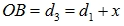Lets also calculate the difference between the travel times:If the plane and the observer were motionless relative to each other, the observer would receive the whole signal wavelength in t0 time. But they are in motion relative to each other. In this case, how long did it take the observer to receive the whole signal wavelength?The equation above gives the length of the signal wavelength that comes to the observer:We can also write the equation as follows:Now, we can form the equations by making use of the characteristics of the Doppler Triangle. Firstly, lets write the Doppler Triangle equations for the OAB triangle. (tΔ= t0):Lets write the distances d1 and d3 with their wavelengths:If we divide the first equation by the second equation: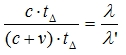Here we reach the final result. While objects are moving away from each other, this equation shows the change on the wavelength.OBTAINING THE  EQUATIONFOR DOPPLER SHIFT FRAMES ARE APPROACHING EACH OTHER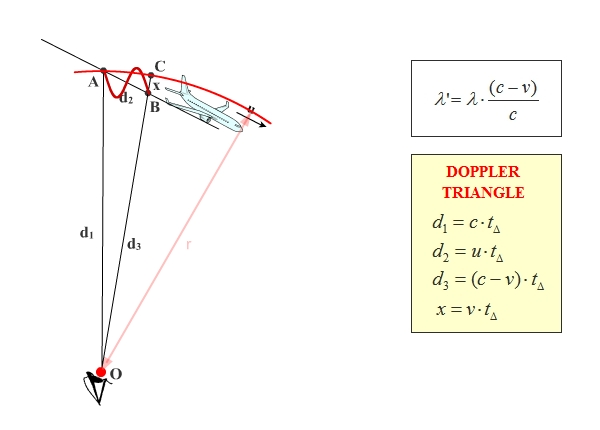The calculations here are completely same as the ones that are done for (c+v). As the similarity is huge, I did not feel the need to write them here as well. However, the OB distance changed here.Because it is, the plus signs are replaced with minuses in relevant parts in the following calculations.In the following parts, the incoming signal wavelength is obtained in this way: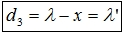When the values are placed in the relevant parts by making use of the Doppler Triangle, the result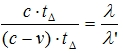is obtained.From here, the final result that shows the wavelength change between the objects that are approaching each other.What is Relativistic Doppler Shift?I felt the necessity to touch upon this topic here. As a consequence of the Relativity Theory, there are many artificially-created equations that have been placed in physics and used up to now. The Relativistic Doppler equation is one of these. Relativistic Doppler is a made-up term that is a product of imagination, and it has no ties with reality. Alice Law eliminated both the Relativity Theory and the term Relativistic Doppler, which is a consequence of the theory.